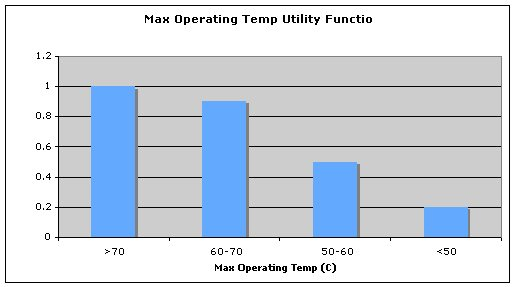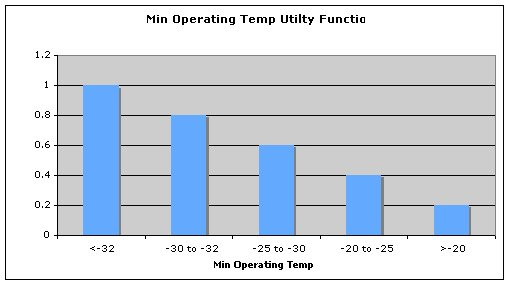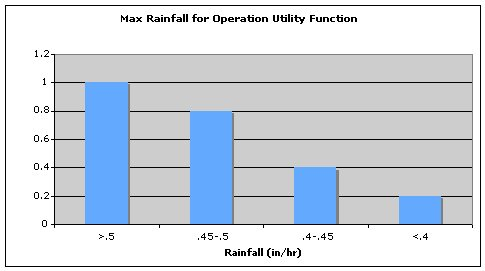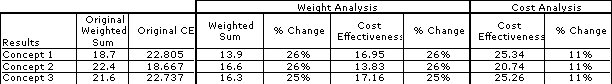Based on the weighted sum evaluation criteria that excluded cost, Concept 2 scored the highest among all the concepts. However, inspecting the results shows that Concept 3, though lower in total sum, still has advantages over Concept 2, especially in accuracy and weight. Upon applying cost analysis into the results, the significantly higher cost of Concept 2 drives the weighted sum/cost well below the other two concepts. Considering both cost and performance, Concept 1 and 3 are very close, with Concept 1 slightly higher. Concept 3 is shown to be an appropriate choice for both evaluations, considering performance alone and considering performance with cost. For this reason, Concept 3 is the most appropriate choice.Sensitivity Analysis

For a sensitivity analysis of weighting, each weight was reduced by one integer to see the impact of a change in weight on the weighted sum and cost effectiveness. With this adjustment, the percent change in the weighted sum was 265 to 26% across the board. Coincidently, the change in weighting values had a similar affect on cost effectiveness, ranging from 25 to 26%. This illustrates that the weighting has a fairly significant affect on the overall score for each alternative.For a sensitivity analysis of cost, each cost was reduced by 10% of its original value to determine the impact of a change in cost to the score, as reflected by the cost effectiveness. The result was that a reduction in cost had an equally significant impact on the cost effectiveness for all three concepts, with an adjustment of 11% on cost effectiveness. If you were able to get a reduction in cost for one of those three companies, it could have a significant impact on the decision.A table of the results of the sensitivity analysis is presented below.Based on the sensitivity analysis performed, Concept 3 remains as the most appropriate option.

Assignment Help | Marketing Assignment Help | Marketing Homework Help | Online Tutoring